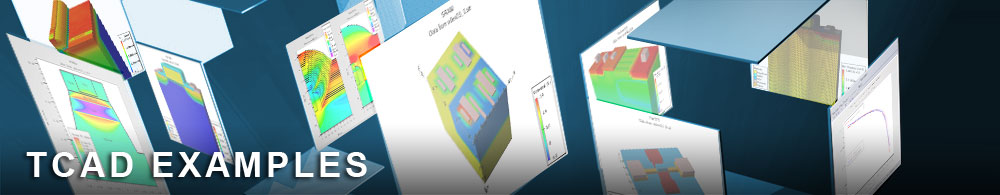Calibration of Electron Bohm Quantum Potential

## quantumex05.in : Calibration of Electron Bohm Quantum Potential

Requires: S-Pisces/Quantum
Minimum Versions: Atlas 5.28.1.R

This example demonstrates:

• Use of the BQP model and Schrodinger-Poisson model on same structure
• Calculation of very low frequency C-V curves using QSCV
• Calibration process

This example illustrates calibration of the Bohm Quantum Potential model against the Schrodinger-Poisson model for an N-MOS capacitor being biased into inversion. Because the calibration takes place under conditions of negligible current flow, the CARRIERS=0 option may be specified on the METHOD statement. The N-MOS has a nominal gate oxide thickness of 20 A. The quantity which was chosen to compare the results of the two models was the quasistatic capacitance. The voltage range was 0V to 1V which includes the depletion to inversion transition region.

The first section calculates the Quasi-static C-V curves using the Schrodinger Poisson model. Note the NUM.DIRECT = 3 specification on the MODELS statement. This ensures that the anisotropic conduction band structure of Silicon is accounted for. Note also that NEW.EIG is also specified on the MODELS statement, this causes a more efficient method to be used to solve the Schrodinger-Poisson equation. QSCV is specified on the solve statement and a fine voltage increment is used in order to give accurate calculation of the Quasi-static Capacitance as a function of voltage. The next three sections calculate the C-V curves over the same voltage range and with the same voltage increment as for the S-P model. Three different sets of BQP parameters are used, the last set gives C-V curves with very good agreement with the S-P results. It is possible that a different set will give even better results, but the ones shown give the same threshold voltage as for S-P and excellent agreement going into inversion. It may take many attempts to obtain close calibration.

To load and run this example, select the Load button in DeckBuild > Examples. This will copy the input file and any support files to your current working directory. Select the Run button in DeckBuild to execute the example.